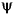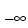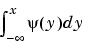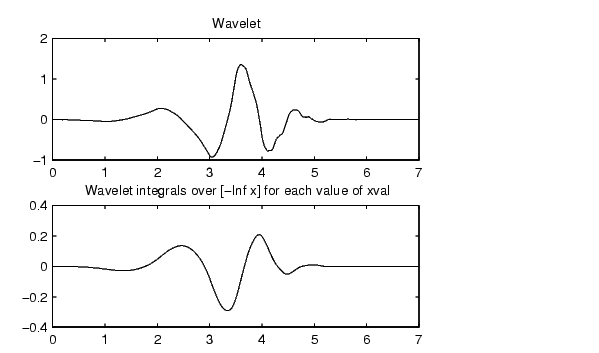Wavelet Toolboxintwave

Integrate wavelet function psi

Syntax

• ```[INTEG,XVAL] = intwave('`wname`',PREC)
[INTEG,XVAL] = intwave('`wname`',PREC,PFLAG)
[INTEG,XVAL] = intwave('`wname`')
```

Description

`[INTEG,XVAL] = intwave(`'`wname`'`,PREC)` computes the integral, `INTEG`, of the wavelet function(fromto `XVAL` values):for x in `XVAL`.

The functionis approximated on the 2PREC points grid `XVAL` where `PREC` is a positive integer. '`wname`' is a string containing the name of the wavelet(see `wfilters` for more information).

Output argument INTEG is a real or complex vector depending on the wavelet type.

For biorthogonal wavelets,

[INTDEC,XVAL,INTREC] = intwave('`wname`',PREC) computes the integrals, INTDEC and INTREC, of the wavelet decomposition functiondec and the wavelet reconstruction functionrec.

`[INTEG,XVAL] = intwave(`'`wname`'`,PREC)` is equivalent to `[INTEG,XVAL] = intwave(`'`wname`'`,PREC,0)`.

`[INTEG,XVAL] = intwave(`'`wname`'`)` is equivalent to `[INTEG,XVAL] = intwave(`'`wname`'`,8)`.

When used with three arguments intwave('`wname`',IN2,IN3), PREC = max(IN2,IN3) and plots are given.

When IN2 is equal to the special value 0,` intwave(`'`wname`'`,0)` is equivalent to `intwave(`'`wname`'`,8,IN3)`.

`intwave(`'`wname`'`)` is equivalent to `intwave(`'`wname`'`,8)`.

`intwave` is used only for continuous analysis (see `cwt` for more information).

Examples

• ```% Set wavelet name.
wname = 'db4';

% Plot wavelet function.
[phi,psi,xval] = wavefun(wname,7);
subplot(211); plot(xval,psi); title('Wavelet');

% Compute and plot wavelet integrals approximations
[integ,xval] = intwave(wname,7);
subplot(212); plot(xval,integ);
title(['Wavelet integrals over [-Inf x] ' ...
'for each value of xval']);```

Algorithm

First, the wavelet function is approximated on a grid of 2PREC points using `wavefun`. A piecewise constant interpolation is used to compute the integrals using `cumsum`.

```wavefun ```ind2depo isnode